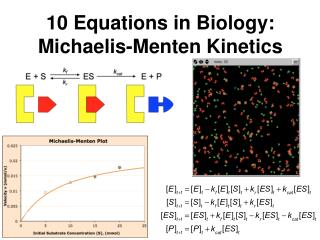Download Presentation10 Equations in Biology: Michaelis-Menten Kinetics# 10 Equations in Biology: Michaelis-Menten Kinetics - PowerPoint PPT Presentation

Download Presentation##### 10 Equations in Biology: Michaelis-Menten Kinetics

Download Policy: Content on the Website is provided to you AS IS for your information and personal use and may not be sold / licensed / shared on other websites without getting consent from its author. While downloading, if for some reason you are not able to download a presentation, the publisher may have deleted the file from their server.

- - - - - - - - - - - - - - - - - - - - - - - - - - - E N D - - - - - - - - - - - - - - - - - - - - - - - - - - -
##### Presentation Transcript

1. 10 Equations in Biology:Michaelis-Menten Kinetics

2. Outline 1. Brief intro to enzyme kinetics 2. From intuitive conceptions to a formal model 3. Building interactive models in Excel 4. From a mathematical model to biological insights

3. Intro to Enzyme Kinetics kf kcat E + S ES E + P kr Variables [E]: free enzyme molecules [S]: free substrate molecules [ES]: enzyme-substrate complexes [P]: free product molecules Parameters kf , kr , kcat : reaction rates

4. \$ in checking account on Sept. 1st = Writing “Word Equations” • A word equation is a qualitative description of the system’s major processes • Goal: help students express their proto-mathematical understanding of a system before introducing formal math. Example: Balancing a checkbook \$ in checking account on Aug. 1st deposits made in August withdrawals made in August ? + –

5. Word Equations for Enzyme Kinetics Model # of free S molecules at time t # of free S molecules binding to free E # of ES complexes splitting into E + S # of free S molecules at time t +1 = – + Write similar word equations for the other 3 variables.

6. Translate Word Equations into Formal Equations # of free S molecules at time t = [S]t conc. of enzyme-substrate complexes # of ES complexes splitting into E + S = kr[ES]t conc. of free enzyme  conc. of free substrate # of free S molecules binding to free E = kf[E]t [S]t by Law of Mass Action

7. Formal Equations for Enzyme Kinetics Model

8. Modeling in Excel Why Excel rather than more powerful tools? • easily available on most campuses • widely used = transferable proficiency • nonthreatening to students • makes students work directly with the equations: no dodges via the user interface!

9. Implementing a Model in Excel ✓ 1. Write a series of recursion equations. 2. Set up Excel sheet with time in Column A, other variables in next columns. 3. Enter and name model parameters; enter variables’ starting values. 4. Enter recursion equations for each variable. 5. Copy and paste for subsequent time steps. 6. Graph results appropriately.

10. Structured Exploration: Understandthe Model 1. How would you measure the reaction’s velocity? How does velocity change over the course of the reaction, and why? 2. How does each rate constant influence the reaction’s velocity? Why?

11. Further Exploration Enzyme Kinetics – Multiple Runs.xlsx • What happens to velocity as [S]0 increases? Why? • Estimatevmax for this enzyme.

12. Further Exploration • Biological interpretation of KM? • Characterize the Michaelis-Menten curves of enzymes w/low, medium, and highKM.

13. Estimating Reaction Constants • What is the shape of the M-M curve? • Nonlinear regression: Fit the appropriate curve to the M-M plot by minimizing the sum of squared residuals. • Use built-in scrollbars to adjust estimates of vmax and Km.

14. Estimating Reaction Constants:Linear Methods Goal: Convert the Michaelis-Menten equation into a form that linearizes the best-fit curve. Example Lineweaver-Burk plot: 1/v vs. 1/[S]0 Formula = …? Should be able to write similar formulas for other plots (H-W, E-H)

15. Estimating Reaction Constants:Evaluating Diff. Methods On what basis might one method be considered “better” than others? • Each method’s sensitivity to estimation error? • Each method’s ability to detect such error?

16. Beyond Simple Enzyme Kinetics How could you modify the model to describe… • Influx or efflux of reaction components? • Reversible, competing, or multi-step reactions? • Totally different biological systems (pop. dynamics, epidemiology, pharmacokinetics, …)?

17. Preview: Agent-Based Models • Simulate & visualize behavior of each indiv. molecule • Focus of next week’s seminar (Nernst equation)

18. Take-home message • Build equations to clarify each term’s meaning and identify key assumptions • Explore models to test understanding of a system, and to ground abstract discussions • Lesson structure: what aspects of this seminar did you find most/least crucial?